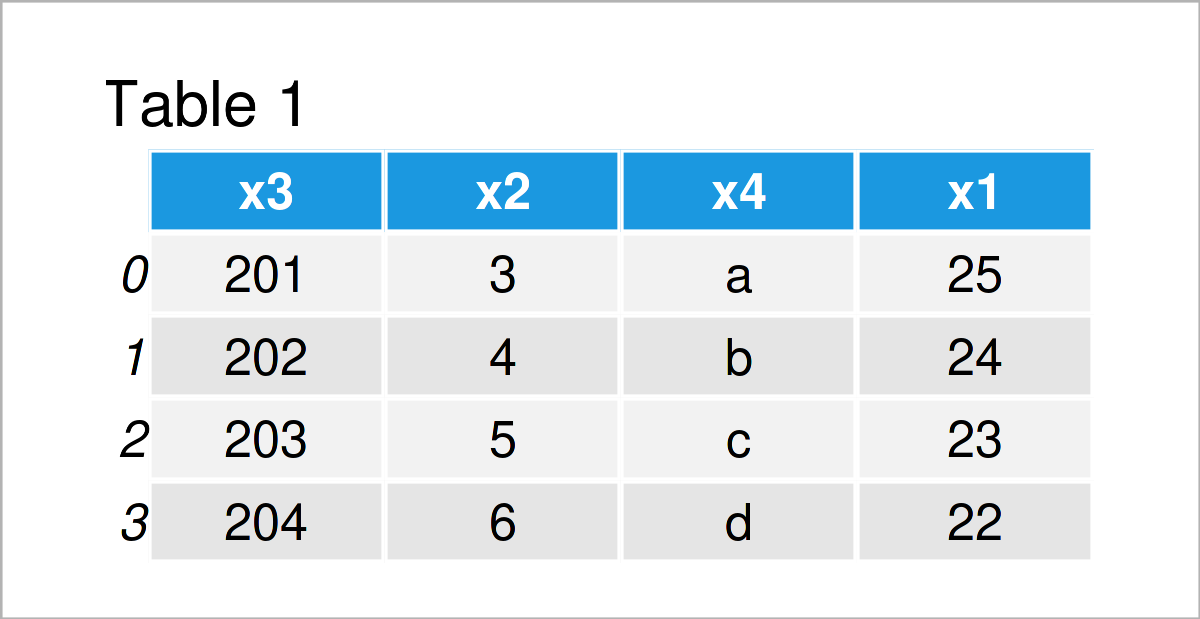# Set Column Order when Writing pandas DataFrame to CSV in Python (2 Examples)

In this Python tutorial you’ll learn how to change the column order when saving a pandas DataFrame as a CSV file.

The tutorial will consist of this:

It’s time to dive into the examples:

## Example Data & Libraries

We first have to import the pandas library, if we want to use the functions that are included in the library.

`import pandas as pd                           # Import pandas library`

As a next step, let’s also create some example data in Python:

```data = pd.DataFrame({'x3':range(201, 205),    # Create pandas DataFrame
'x2':range(3, 7),
'x4':['a', 'b', 'c', 'd'],
'x1':range(25, 21, - 1)})
print(data)                                   # Print pandas DataFrame```As you can see based on Table 1, our exemplifying data is a pandas DataFrame containing four observations and four columns. The order of these columns is currently set to x3, x2, x4, and x1.

## Example 1: Keep Column Order when Saving pandas DataFrame as CSV File

Example 1 explains how to preserve the column order when writing a pandas DataFrame to a CSV file.

In newer versions, this is fortunately the default specification of the to_csv function. For that reason, we don’t have to assign any additional settings:

`data.to_csv('data1.csv')                      # Export pandas DataFrame`As illustrated in Table 2, the previous Python code has created a new CSV file containing exactly the same variable sorting as in our input data set.

## Example 2: Change Column Order when Saving pandas DataFrame as CSV File

In this section, I’ll illustrate how to manually adjust the ordering of the columns of a pandas DataFrame when exporting this DataFrame to a CSV file.

For this task, we have to specify a list containing the desired column order to the columns argument within the to_csv function:

```data.to_csv('data2.csv',                      # Export pandas DataFrame
columns = ['x1', 'x2', 'x3', 'x4'])```The output of the previous syntax is shown in Table 3 – Another CSV file output containing a user-defined ordering of the columns.

## Video, Further Resources & Summary

Have a look at the following video on my YouTube channel. I’m explaining the Python programming codes of this page in the video.

In addition to the video, you could read the other articles that I have published on this homepage.

To summarize: This tutorial has demonstrated how to set the variable order when saving a pandas DataFrame as a CSV file in the Python programming language. In case you have any further questions, please let me know in the comments below.

Subscribe to the Statistics Globe Newsletter